## RR's Unit Converter Help

User Interface

Search Interface

Algebraic Calculations

Mathematical formulas and constants

Settings

### User Interface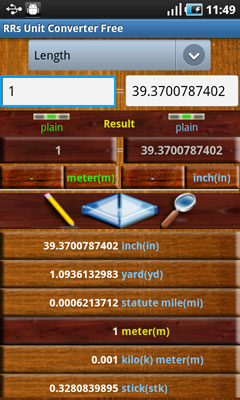Category selection spinner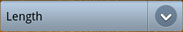Click this spinner to select unit categories.

Edit boxes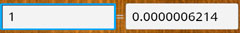Input and Output edit boxes. Click to enter your data to be converted. From input to output if input is selected or vice versa.

Result boxes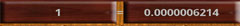Result boxes. Displays convertion result or algebraic expresions parsing output.
Two more actions are attached to those boxes:
Short Click - with each click will change number format by sequentially passing through three formats available:
- auto;
- plain;
- engineering;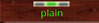Shows what number format is selected.

Long Click - available only when a compound unit is selected. Will popup 'Edit denominator' dialog box.

Unit Selectors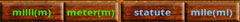Unit selectors for input( green) and output( blue) units.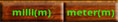Each selector is split in two.
First box will select derivates or prefixes of the main unit which can be selected by using the second box.
Actions available:
Short Click - opens units or prefixes/derivates list to select from.
Long Click - will open 'Select Menu' with two options:
- Add to watchlist( only available for simple units);
- Show definition;

Buttons bar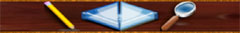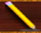Pencil button:
Short click - will open mathematical formulas list.
Please view Mathematical formulas section for more details about what formulas are available.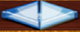Switch button, has two functions:
Short click - switch input with output units.
Long click - switch watchlist mode. Two modes are available:
- input to many( all items in watchlist);
- many to output;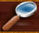Search button:
Short click - opens search unit interface.

WatchList
Watchlist in input to many mode: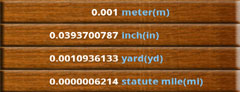Watchlist in many to output mode: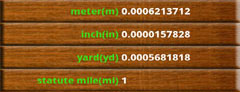### Search Interface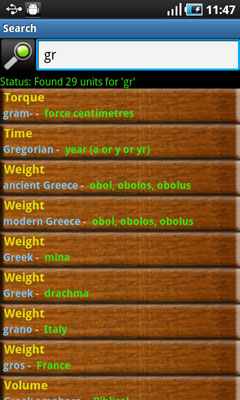Into the edit box type the name or only partial name for the unit(s) you are looking for.
Search result will be displayed as a list.
Colors meneanigs: Categories, Prefixes or Derivates, Units
Long Click an element from the list and you will be returned to program main screen with the unit selected as input.

### Algebraic Calculations

Use simple arithmetic symbols like +, -, *, /, (), to make calculations directly in RRs Unit Converter.
Screen shot to demonstrate this: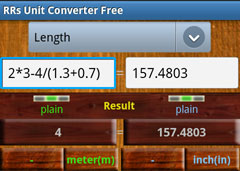### Mathematical formulas and constants

For your convenience RRs Unit Converter has some built in mathematical formulas and constants.
Access those formulas by using 'Pencil Button'
Constants:
• e() - constant e number
• pi() - constant pi number

Geometric Formulas:
• Area:
• Rectangle
• Parallelogram
• Triangle
• Trapezoid
• Circle
• Volume:
• Rectangular Solid
• Prisms
• Cylinder
• Pyramid
• Cones
• Sphere

Mathematical formulas:
• rand() - generates random number
• sin(x)
• cos(x)
• tan(x)
• asin(x)
• acos(x)
• atan(x)
• asinh(x) → 2 * ln(sqrt((x+1)/2) + sqrt((x-1)/2))
• acosh(x) → ln(x + sqrt(1 + x2))
• atanh(x) → (ln(1+x) - ln(1-x)) / 2
• sinh(x) → (ex - e-x)/2
• cosh(x) → (ex + e-x)/2
• tanh(x) → (ex - e-x)/(ex + e-x)
• sqrt(x) → Math.sqrt(double)
• abs(x) → Math.abs(double)
• ceil(x) → Math.ceil(double)
• floor(x) → Math.floor(double)
• exp(x) → ex
• ln(x) → logex
• lg(x) → log2x
• log(x) → log10x
• sign(x) → x > 0 = 1, x < 0 = -1, else 0
• fact(n) → n! = 1 * 2 * ... * (n - 1) * n
• round(x) → Math.round(double)
• log(x,y) → logyx
• combin(n, r) → PascalsTriangle.nCr(n, r)
• mod(x, y) → x % y
• pow(x, y) → xy
• min(x1,x2,...,xn)
• max(x1,x2,...,xn)
• sum(x1,x2,...,xn) → x1 + x2 + ... + xn
• avg(x1,x2,...,xn) → (x1 + x2 + ... + xn) / n

### Settings

The following settings are available:
• Precision:
Set calculation precision used by RR's Unit Converter during calculations.
RR's Unit Convertor uses an infinite-precision arithmetic alghoritm. So, it's limited only by your device memory.
• Watchlist Sort Order Select sort order for watchlist( ascendent or descendent). Watchlist will be sorted only by insert history.
• Search list sort
• Sort by search result.
You may sort search results by Category Name, Unit Name, Prefixes/Derivates Name or Find order.
• Sort order: Ascendent or Descentent
• Keyboard Layout:
Select virtual keyboard layout used for editing unit value.
• Hide Denominator:
Select this if you want to hide denominator if it equals to 1.